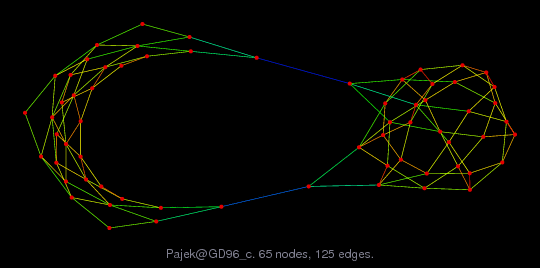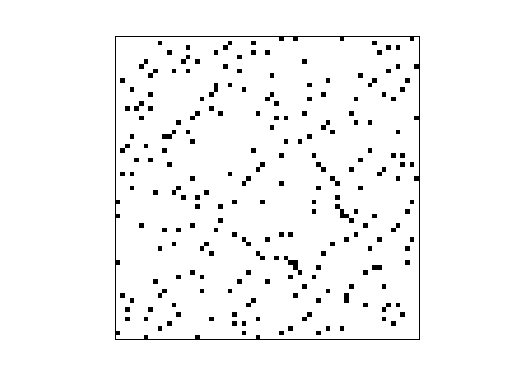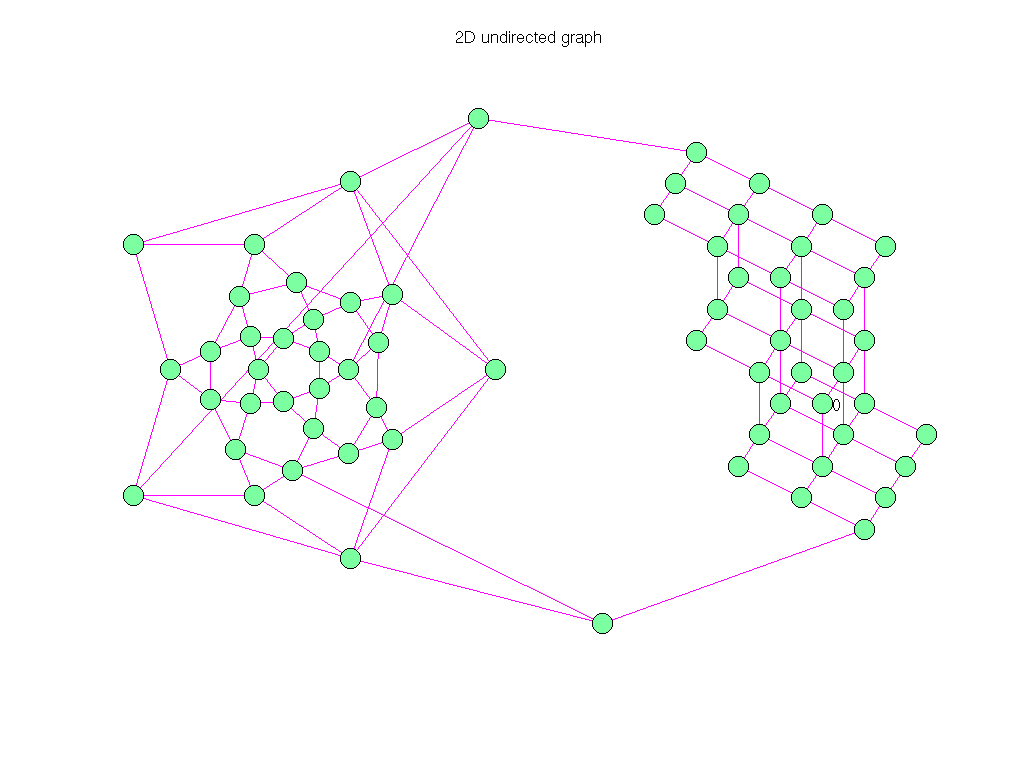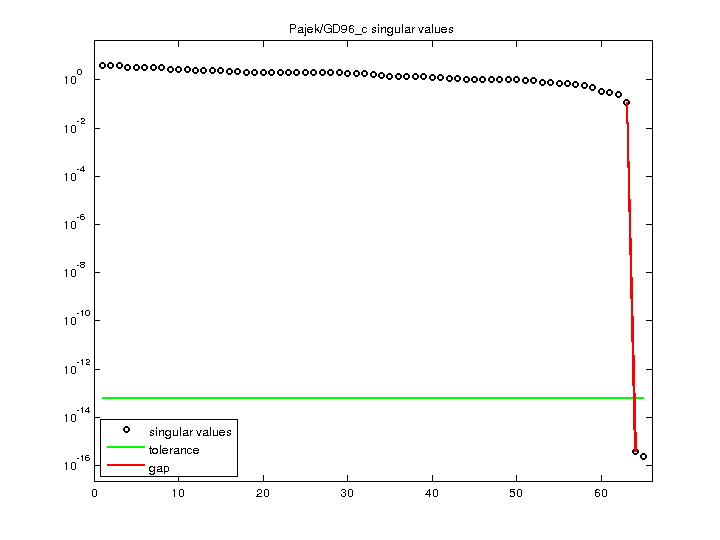Matrix: Pajek/GD96_c

Description: Pajek network: Graph Drawing contest 1996(undirected graph drawing)• Matrix group: Pajek
• download as a MATLAB mat-file, file size: 2 KB. Use UFget(1490) or UFget('Pajek/GD96_c') in MATLAB.

 Matrix properties number of rows 65 number of columns 65 nonzeros 250 # strongly connected comp. 1 explicit zero entries 0 nonzero pattern symmetry symmetric numeric value symmetry symmetric type binary structure symmetric Cholesky candidate? no positive definite? no

 author Graph Drawing Contest editor V. Batagelj date 1996 kind undirected graph 2D/3D problem? no

 Additional fields size and type nodename full 65-by-1 coord full 65-by-2

Notes:

```------------------------------------------------------------------------------
Pajek network converted to sparse adjacency matrix for inclusion in UF sparse
matrix collection, Tim Davis.  For Pajek datasets, See V. Batagelj & A. Mrvar,
------------------------------------------------------------------------------
The original problem had 3D xyz coordinates, but all values of z were equal
to 0, and have been removed.  This graph has 2D coordinates.
```

 SVD-based statistics: norm(A) 4.14108 min(svd(A)) 2.28069e-16 cond(A) 1.81572e+16 rank(A) 63 null space dimension 2 full numerical rank? no singular value gap 2.97374e+14

 singular values (MAT file): click here SVD method used: s = svd (full (A)) ; status: ok